Next: Sources of Multipole Radiation Up: Multipole Expansion Previous: Properties of Multipole Fields

# Solution of Inhomogeneous Helmholtz Equation

The inhomogeneous Helmholtz wave equation is conveniently solved by means of a Green's function,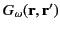, that satisfies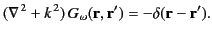(1506)

The solution of this equation, subject to the Sommerfeld radiation condition, which ensures that sources radiate waves instead of absorbing them, is written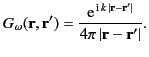(1507)

(See Chapter 1.)

As is well known, the spherical harmonics satisfy the completeness relation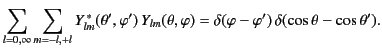(1508)

Now, the three-dimensional delta function can be written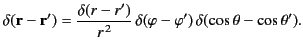(1509)

It follows that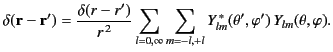(1510)

Let us expand the Green's function in the form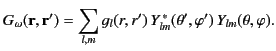(1511)

Substitution of this expression into Equation (1508) yields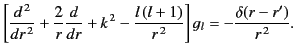(1512)

The appropriate boundary conditions are that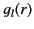be finite at the origin, and correspond to an outgoing wave at infinity (i.e.,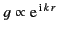in the limit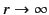). The solution of the above equation that satisfies these boundary conditions is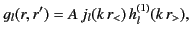(1513)

where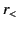and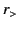are the greater and the lesser of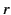and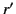, respectively. The appropriate discontinuity in slope at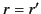is assured if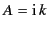, because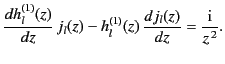(1514)

Thus, the expansion of the Green's function becomes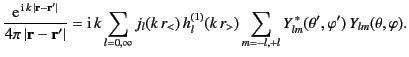(1515)

This is a particularly useful result, as we shall discover, because it easily allows us to express the general solution of the inhomogeneous wave equation as a multipole expansion.Next: Sources of Multipole Radiation Up: Multipole Expansion Previous: Properties of Multipole Fields
Richard Fitzpatrick 2014-06-27